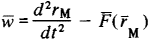(redirected from inertial guidance system)
Also found in: Dictionary, Thesaurus, Acronyms, Wikipedia.
Related to inertial guidance system: inertial platform, inertial navigation system

[in′ər·shəl ‚nav·ə′gā·shən ‚sis·təm]
A self-contained system that can automatically determine the position, velocity, and attitude of a moving vehicle by means of the double integration of the outputs of accelerometers that are either strapped to the vehicle or stabilized with respect to inertial space. Also known as inertial navigator.

a navigation device whose operation is based on the classical (Newtonian) laws of mechanics. In an inertial navigation system an inertial system (an absolute system, that is, one that is stationary with respect to the stars) is the initial (main) frame of reference, with respect to which inertial measurements are made. The coordinates, velocity, and acceleration of an object, such as an aircraft, missile, spacecraft, or surface or submerged vessel, are determined by means of an inertial navigation system. Such systems have major, important advantages over other navigation systems— their universality of application, the possibility of determining the main parameters of motion, autonomy of action, and absolute noise resistance. These qualities have made inertial systems the most promising type of navigation system.

The principle of operation of inertial navigation systems consists in the simulation (representation) of the translational motion of an object, characterized by the change with time in the acceleration, velocity, and coordinates, which is similar to the process of motion of the sensing element (mass) of a three-dimensional (three-component) accelerometer (which, in the general case, has compensation for gravitational acceleration). The equation of motion of the sensing element in the inertial coordinate system is the main equation of the inertial method of determining the parameters of motion; in the general case it has the formwhere w is the acceleration measured by the accelerometer, M i is the radius vector of the point M (the center of gravity of the sensing element) in the inertial coordinate system, is the force of attraction of a unit mass of the sensing element at the point M (the acceleration of gravity).

The essence of the inertial method is the measurement with an accelerometer of the initial parameter (acceleration) and the integration of the main equation—single integration to determine the velocity and double integration to determine the coordinates. The measuring axes of the accelerometers are oriented in preset directions by means of gyroscopic devices that are free or controlled (by signals from the accelerometers), such as a gyroscope, gyrostabilizer, or gyroframe, or by astrostabilizers, as well as by a combination of methods. Gyroscopic and electromechanical integrators are used to integrate the main equation. An inertial navigation system contains a plotter (an inertial vertical) or a computer of the direction of the local vertical. An inertial vertical has high precision and is not disturbed—that is, does not deviate from the local vertical—under horizontal accelerations.

Inertial navigation systems are classified according to a number of features, such as the orientation of the axes of sensitivity of inertial gauges (arbitrary orientation, orientation relative to the stars, orientation with respect to axes rigidly connected to the object, constant orientation with respect to a celestial body such as the earth, or horizontal orientation), the method of plotting the local vertical (with an analytical, or calculated, vertical or with an inertial vertical plotter), and the presence of a stabilized platform (with or without a stabilized gyroscope or astroplat-form).

Inertial navigation systems are extremely complex and costly. Their service life is less than that of ordinary gyroscopic instruments. To ensure correct functioning of an inertial navigation system, initial data on the coordinates of the launch site and velocity must be entered prior to launch, and the inertial gauges must be oriented. The accuracy of uncorrected inertial navigation systems depends on the time. Therefore, the possibility of obtaining data from an inertial navigation system that satisfy the given requirements is limited in time. In flight the best types of inertial navigation systems have an error of approximately 1.5–5.0 km in the determination of coordinates. Various methods of correction by using radio-navigation, radar, and astronavigation devices are used to reduce errors and expand the possibilities for use.

REFERENCES

Printsipy inertsial’noi navigatsii. Edited by V. A. Bodner. Moscow, 1965. (Translated from English.)
Pomykaev, I. I. Inertsial’nyi metod izmereniia parametrov dvizheniia letatel’nykh apparatov. Moscow, 1969.

I. I. POMYKAEV